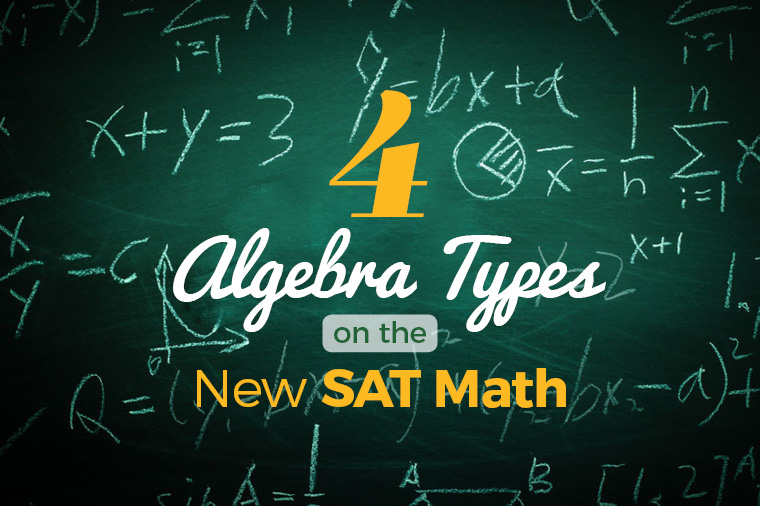## 4 Types of Algebra Based Questions Common to the New SAT Test4 Types of Algebra Based Questions Common to the New SAT Test

The new SAT Math comes with many changes. One of those is increased focus on algebra based questions (see our previous post The New SAT Math Easy or Hard?). So how should you prepare for these questions?

Even though algebra based questions could look similar, each of them is likely to test a different area of knowledge. These 4 types of questions are most common in the New SAT Math:

• Algebraic Expressions
• Linear Equations
• Inequalities
• Functions
1. Algebraic Expressions

Algebraic expressions are the most fundamental aspect of algebra. In order to understand algebraic expressions, you must be familiar with constants, variables, coefficients, and operators. For example, in this equation

### 6 x + 10

the number 10 is a constant, 6 is a coefficient, x is a variable, and the plus sign is an addition operator. Note that there is also an implicit multiplication operator between 6 and the variable x. Other than the elements of an algebraic expression, make sure you are also familiar with the algebraic properties, namely the commutative, associative, and distributive properties.

Click here for our video tutorial on how to combine like terms using algebraic properties.

2. Linear Equations

Linear equations build upon the idea of algebraic expressions by adding an equality into the statement. Essentially, linear equations are mathematical statements that equate two algebraic expressions. Here are some examples of linear equations:

### 5 x + 8 = 9 + x

You would most likely need to manipulate the linear equations in order to isolate and solve for the desired variable. The most common techniques in solving linear equations are: adding/subtracting both sides of the equation by same amount, multiplying/dividing both sides of the equation by same amount, and expanding/consolidating terms.

Click here for our video tutorial on how to solve linear equations.

3. Inequalities

Just like linear equations, inequalities also build upon algebraic expressions, but by adding an inequality rather than an equality. Here are some examples:

### | 2 x – 4 | < 8

While you would also manipulate the algebraic expressions on both sides of the inequality in order to isolate the desired variable, there is one complication that you must watch out for: multiplying/dividing both sides of the equation by a negative number would result in a flipped inequality sign (i.e. > would become <, and vice versa). Also, you could encounter inequalities involving absolute values, and these could seem difficult at first. However, there is a systematic way to solve absolute inequalities. If the absolute value, which contains the variable, is less than a constant, the solution is a single range, where both the lower and upper bounds are defined. If the absolute value is greater than a constant, the solution is two disjoint ranges, where one range has a defined lower bound and an infinity as the upper bound, and the other range has a defined upper bound and a negative infinity as the lower bound.

Click here for our video tutorial on how to solve a basic inequality question, and here for another tutorial on how to solve absolute inequality.

4. Functions

We have already talked about linear equations, and they are a type of function. Other functions you are likely going to encounter on the SAT Math are quadratic functions and trigonometric functions. Here are some examples:

### g(x) = 2 sin(x) + cos (x)

So what are functions? The most intuitive explanation is that a function illustrates a relationship. For example, a function could show the relationship between a company’s sales revenue and the number of units sold, and this relationship can be graphed as well. In the graph of a function, a single input value can only have a single output value, since a function illustrates a one-to-one relationship. The notations f(x), g(x), h(x), etc, which all read “function of x”, are the same as the symbol y which you are probably more familiar with in an equation. Whenever you see something like f(3) in a question, simply substitute all symbols x in the equation with the number 3. Also, make sure you are familiar with the concepts of domain and range. Domain is the set of values that the input (often denoted x) can take, whereas the range is the set of values that the output (often denoted f(x)) can take.

Click here for our video tutorial on functions and factors, and here for our tutorial on trig functions.

Once you tackle these four most common types of algebra based questions, you are well on your way to acing the SAT Math. Good luck!